Communicate with Supplier? SupplierMs. Jane Deng
What can I do for you?

Industry Information

Home > Industry Information > Is the constant voltage power supply or the constant current power supply used to power the LED?

# Is the constant voltage power supply or the constant current power supply used to power the LED?

2019-10-17

Shanghai Longmao Microelectronics Co., Ltd. Mao Yuhai

So, should we use a constant voltage power supply or a constant current power supply?

Let's first look at what kind of device the LED is. Because the brightness of the LED is proportional to its forward current, and the structure of some LEDs determines its heat dissipation is the power consumption. Therefore, most LEDs will give the rated current, for example, Φ5 is 20mA, 1W is 350mA...etc. But this does not mean that the LED can only work at these rated currents, and it does not mean that the LED is a constant current device. For example, Cree's 1 watt LED and 3 watt LED are the same model, the current is increased from 350 mA to 700 mA, and the power is increased from 1 W to 3 W, so this LED can work at any value between 350-700 mA.

To understand this problem in depth, you must first understand the volt-ampere characteristics of LEDs.

1. Volt-ampere characteristics of LED

The Chinese name of the LED is the LED, so it is a diode itself. Its volt-ampere characteristics are very similar to the general diode volt-ampere characteristics. But usually the curve is very steep. For example, the volt-ampere characteristics of a 20 mA straw hat LED are shown in Figure 1.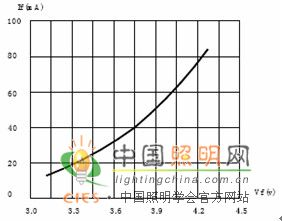Figure 1. Voltammetric characteristics of low power LEDs

If it is powered by a dry battery or a battery, because of the nonlinearity of the volt-ampere characteristics of the LED, a small voltage change will cause a large current change. In the above figure, the forward voltage is 20mA when the power supply voltage is 3.3V. Three sections of dry battery power supply, the new battery voltage exceeds 1.5V, the three sections are 4.5V, the LED current will exceed 100mA, and will burn out soon. The same is true for 1W high-power LEDs. Figure 2 shows the volt-ampere characteristics of a company's 1W LED. The voltage of a 12V battery can be reduced from 14.5V to 10.5V when fully charged to fast-release. The difference is nearly 20%. As can be seen from the volt-ampere characteristics, a 10% change in the supply voltage (3.4V-3.1V) causes a 3.5-fold change in the forward current (from 350mA to 100mA).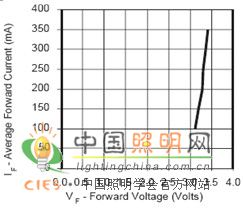Figure 2. Voltammetric characteristics of a 1W high power LED

2. Temperature coefficient of volt-ampere characteristics

Until now, many people think that the LED voltage is fixed, and the current is fixed, so the constant voltage and constant current are the same. In fact, the volt-ampere characteristics of LEDs are not fixed, but vary with temperature, so the voltage is fixed, the current is not necessarily, but varies with temperature. This is because the LED is a diode whose volt-ampere characteristics are characterized by a negative temperature coefficient.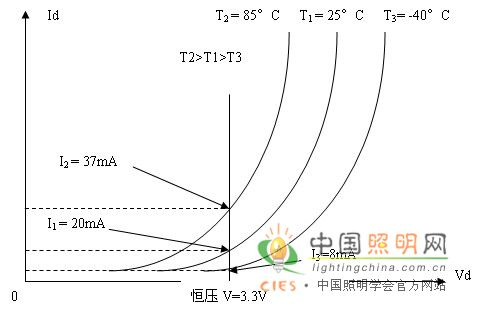Figure 3. Temperature characteristics of volt-ampere characteristics

The temperature coefficient is usually -2 mV / deg (-1.5 - 2.5 mV / ° C), that is, as the temperature increases, its volt-ampere characteristics shift to the left, if the applied voltage is constant, then the current will increase. The efficiency of the LED itself is very low, and the temperature rise is very high. After power-on, if the heat dissipation is not good, the temperature can easily rise to eight or ninety degrees. It is assumed that a 3.3V constant voltage source operates at 20 mA at normal temperature, and when the temperature rises to 85 degrees, the current increases to 35-37 mA, and its brightness does not increase. An increase in current will only increase its temperature rise, which will increase light decay and reduce life.

Moreover, if a constant voltage source is used instead of a constant current source, when operating at 20 mA at normal temperature, the current will decrease to 8-10 mA at -40 degrees, and the brightness will decrease.

For the 1W high-power LED chip, the situation is the same, and because of the large power, the heat dissipation is not easy, and the temperature rise problem is more serious.

It can be said that in addition to the heat dissipation problem, the use of constant voltage power supply is the main cause of light decay. Therefore, in principle, LEDs are prohibited from being powered by a constant voltage power supply.

3. Can I stabilize the current with a series resistor after using a constant voltage power supply?

The series resistor only has a finite current effect, that is, if the power supply voltage is higher than the voltage after the LED is connected in series, then a series resistor is needed to limit the current to avoid damaging the LED. However, if you want to use series resistors to reduce the effect of temperature, its role is very small, which can be seen from the volt-ampere characteristics, the series resistance can indeed reduce the current rise caused by temperature rise, the more the resistance Large, the current changes with temperature is smaller, but only decreases, and can not be eliminated. And it is clear that the resistor will bring extra power consumption, making the overall efficiency of the LED lower. Assume that the LED used is a 1W LED with a current of 0.35A. Assuming that the series resistance is 100 ohms, it is obviously unacceptable to consume as much as 12.25 W. Even if the resistance is reduced to 10 ohms, the power consumption is still 1.225 W. More power than the LED itself. In order to reduce this power consumption, it is necessary to reduce the resistance again. However, the result of reducing the resistance is such that the current change caused by the temperature rise is still increased. Therefore, series resistance is by no means a good solution.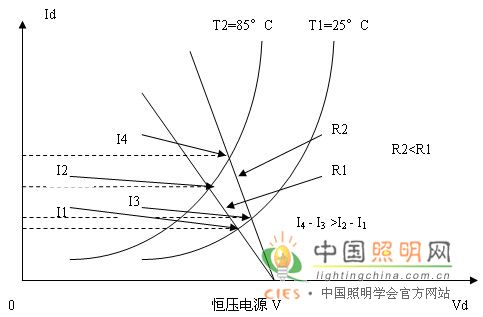Figure 4. Series resistance can only reduce the effect of temperature, but not its effect.

4. Several LEDs are connected in parallel. Can you use a constant voltage power supply?

Due to the discrete nature of the volt-ampere characteristics of LEDs, not only the volt-ampere characteristics of the same wattage LEDs produced by different manufacturers are different, but the volt-ampere characteristics of the same type of LEDs produced by the same manufacturer are also different.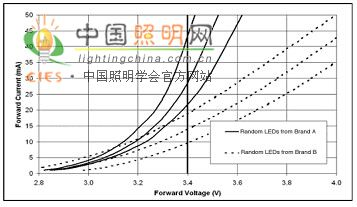Figure 5. Discreteness of volt-ampere characteristics of LEDs produced by different manufacturers and the same manufacturer

Obviously, if you use a constant voltage power supply of 3.4V, it is obvious that the current flowing through each LED is different, and the brightness of each LED is different. Therefore, it cannot be powered by a constant voltage power supply.

5. After multiple LEDs are connected in parallel, use constant voltage power supply. Can you use different series resistors to balance the current?

It is ok at normal temperature, but it cannot be maintained after the temperature rise. This problem is shown in Figure 6. The volt-ampere characteristics of the LED at normal temperature are indicated by solid lines. The volt-ampere characteristics of the two LEDs are slightly different in slope. When using the constant voltage power supply Vo, different resistors can be used. The same forward current Io is obtained. However, when the temperature rises, its volt-ampere characteristics shift to the left, as indicated by the dotted line. Because it is still the original constant voltage and the original resistance, the current at this time becomes I1 and I2. Not equal to the original Io.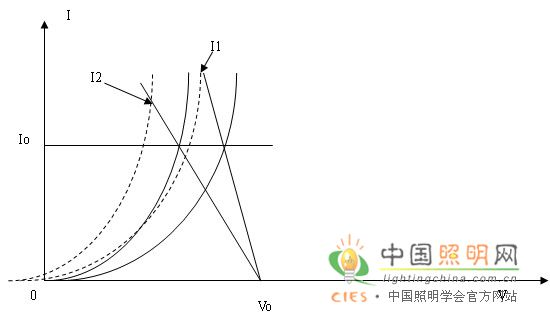Figure 6. Series resistors keep their current constant at room temperature, but they do not maintain current balance after temperature rise.

6. After the N LEDs are connected in series, if the power is supplied by the constant voltage power supply, the temperature effect (the current increase caused by the temperature rise) will be expanded by N times. This is because all the LEDs are connected in series to be equivalent to the volt-ampere characteristic of each LED. Voltage axis in series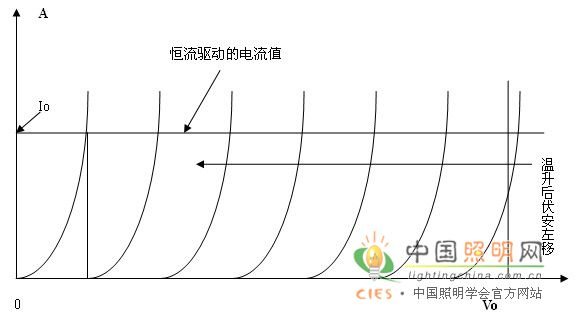Figure 7. Multiple LEDs in series, equivalent to multiple volt-ampere characteristics stacked at a constant current point. After power-on, the temperature rises and all volt-ampere characteristics shift to the left.

After the temperature rise, the N volt-ampere characteristics are shifted to the left, and the current increase is also increased by N times. If the constant current power supply is used, the temperature can be kept constant at Io after the temperature rise.

7. When multiple LEDs are connected in series, when using constant current power supply, the temperature effect of volt-ampere characteristics can be used to estimate the degree of rise of junction temperature.

In many applications (such as fluorescent lamps, street lamps), many LEDs are often connected in series. At this time, the temperature coefficient effect of the LED is more obvious. Because the power is supplied by a constant current power supply, the effect is equivalent to superimposing the volt-ampere characteristics of each LED along the voltage axis. If the temperature rise is 60 degrees, the volt-ampere characteristic will be shifted to the left by 0.12V. If 10 LEDs are connected in series, all volt-ampere characteristics will shift to the left and the total offset will reach 1.2V. This is a considerable number.

In turn, the LED can be used to measure its junction temperature. For example, there is a 10-string 3-in combination of LEDs. After the constant current source is connected, the forward voltage drop is reduced from 32.3V to 30.6V. The change is up to 1.7V. Then it can be speculated that the junction temperature rises to 1.7/10/.002=85 degrees.

8. How to ensure current balance of each string in series-parallel circuit when constant current supply

If only a string of LEDs is supplied with a constant current source, then of course it is ideal. However, if you want to supply several strings of parallel LEDs, how can you guarantee the same current in each string?

Yes, if a series of parallel LEDs are supplied with a constant current source, the current of each string must be different due to the discrete nature of the volt-ampere characteristics of the LED. But in fact, because each string of LEDs is unlikely to have a low forward voltage in one string, the forward voltage is high in the other string. Rather, it will be relatively evenly distributed, so the currents between the strings will not vary greatly.

9. How to avoid problems caused by damage of a certain LED in a series-parallel circuit with constant current supply

If only two strings are connected in parallel, and one of the LEDs of one of the strings is broken (open circuit), not only will the string not light up, but all the current will flow to the other string, causing the current of the other string to double. It won't take long to break. In order to avoid a broken string of light, then all and parallel methods can be used, that is, each of the strings is connected in parallel with the LEDs of the same position in the other strings. In this way, any one is broken (open circuit), but this one is not bright, and the remaining LEDs are still lit. But if there are only two strings of LEDs in parallel, one of the LEDs is open, the current flows to the other LED in parallel with it, and its current is doubled, so that the life of this LED is not long, very soon. Burning off; if the burnout is an open circuit, it will cause all the LEDs to be off, but the other LED damage is not serious, because there is no long-term operation in the overcurrent state. In order to reduce the influence of one LED on other LEDs after damage, it is desirable that the number of LED strings connected in parallel is as good as possible. In Fig. 7, three strings of 5 are shown and the figures are merged. At this time, one of the LEDs is broken, the total current is distributed to the remaining four LEDs, the total current is distributed among all the parallel LEDs in each row, and the current divided by the low forward voltage LED is larger. But it will not cause too much harm.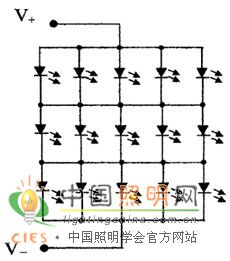Figure 8. Each of the three strings is connected in parallel with the LEDs in the same position in the other strings.

And just the current in this line is divided into the remaining 4, while the other lines are still the same. If the LED is short-circuited instead of open, the other LEDs in this row will not light up.

Of course, in order to avoid this phenomenon, the best way is to connect a voltage regulator tube in parallel with each LED, and do not connect the strings in parallel. At this time, any LED is broken (open circuit), the Zener diode is turned on, and the current distribution relationship changes little. A short circuit is one less LED illumination.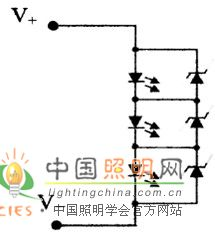Figure 9. Each LED is connected in parallel with a Zener

After adopting this method, there is no need to parallel the parallel.

Summary The above description can be listed as follows:

 Knot Structure Any one is broken (open circuit) Any one is broken (short circuit) a separate string This series of open circuits is not bright This one is not bright Two strings in parallel This string is not bright, the other string of all LED current doubles, the life is shortened, and it is soon bad. This one is not bright, this string of current is slightly increased Two strings in parallel This one is not bright, another current in the same line doubles, and it quickly breaks down; if it is open circuit, all does not light, such as the short circuit, the two LEDs are not bright. This one is not lit, and the other in parallel with it is not bright. N string parallel connection This one is not bright, and the rest of the current is increased by N/ ( N-1 ) times. The bigger N is the safer This one is not bright, and the other ones in parallel with it are not bright. Each LED parallel regulator tube, each series and parallel Only this one is not bright, and has little effect on others. Just this one is not bright

So isn't the constant voltage power supply useless in LED lighting? This is not the case at all.

10. In the mains LED street light, the method of constant voltage Switching Power Supply plus constant current module is used to supply power.

An AC/DC switching power supply is required in any utility-powered system. There are two ways to supply power. One is to add a constant current feedback control circuit to the switching power supply to ensure a constant output current. However, most of this method can only output a single large current, and the accuracy of the constant current is not high. Another is that the front is a constant voltage power supply, followed by a lot of constant current modules. This solution has high flexibility and high constant current accuracy. For example, a 150W-300W mains LED street light power supply scheme is as follows: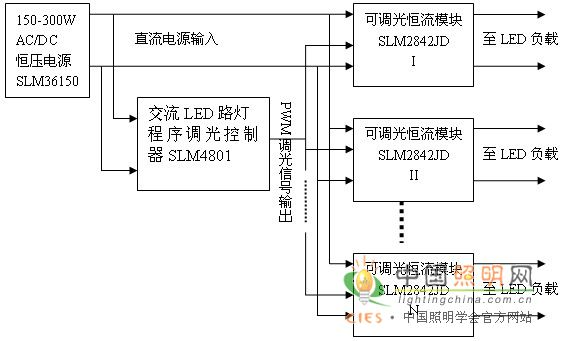Moreover, the biggest advantage of this structure is that it can be programmed to dim, saving energy by more than 40% .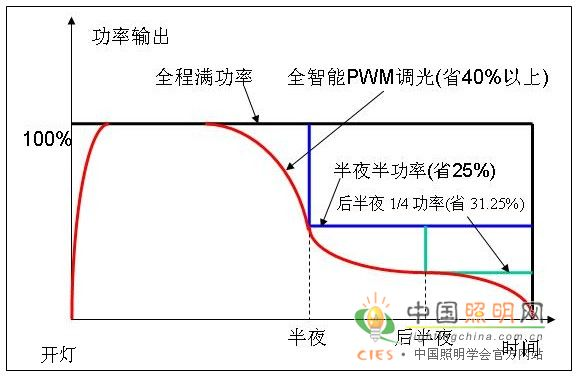Because it is beyond the scope of this article, I will not say more here.

(Author's contact method: yuhai., company website: )

Send InquiryMs. Jane Deng

Tel:86-132-66660104

Fax:86-0755-81461215

Mobile Phone:

Email:power15@szzhpower.com

Address:8-9 Floor, Building 2, Fengxing Lane No.1, Fenghuang First Industrial Zone, Fuyong St., Baoan Dist., Shenzhen, Guangdong, China, Shenzhen, Guangdong

Mobile Site